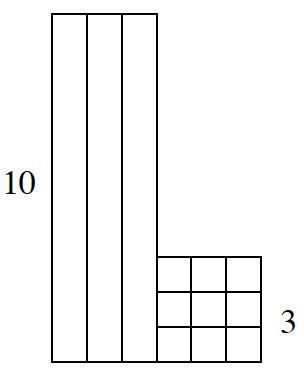### Home > CC1 > Chapter 3 > Lesson 3.1.6 > Problem3-88

3-88.

The diagram at right is made up of Base Ten Blocks. Use the diagram to answer the following questions.

1. Find the area and perimeter of the shape.

The area is the space inside the drawing.The perimeter is the length around the edges.

2. Draw a Base Ten Block shape with a value of $126$ using the fewest number of blocks possible. Find the perimeter of the shape that you drew.

There are $9$ blocks if you use the fewest blocks possible.Use the eTool below to find the area and perimeter of the shape and model the new shape.
Click the link at right for the full version of the eTool:
3-88 HW eTool